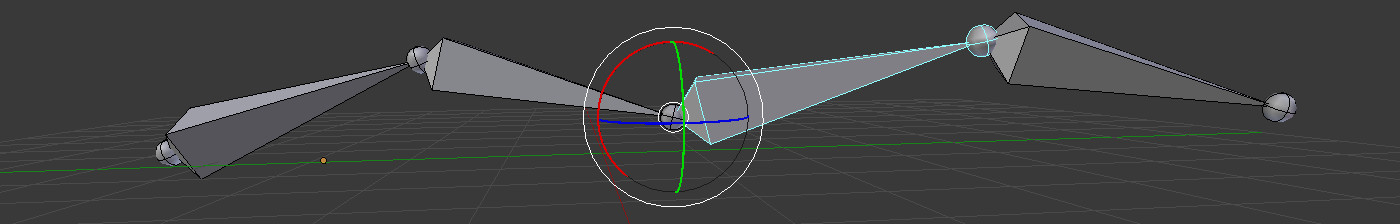# Skeletal animation, forward kinematic## Linear Blending Skinning (LBS) formula

$$\begin{equation*} \bar{\mathbf{p_i}} = \sum_{j=1}^{n} w_{ij} T_j \mathbf{p_i} \end{equation*}$$

• $$n$$ number of bones
• $$w_{ij}$$ scalar weight at the i$$^{\text{th}}$$ vertex associated to the j$$^{\text{th}}$$ bone
• $$T_j$$ the 4x4 matrix, the global transformation of the j$$^{\text{th}}$$ bone from its rest pose.
• $$\mathbf{p_i}$$ mesh's vertex in rest pose
• $$\bar{\mathbf{p_i}}$$ the vertex after deformation.
Vec3 linear_blending_skinning(
const std::vector< std::map<int, float> >& skinning_weights,
const std::vector<Mat4x4>& skinning_transfos,
const std::vector<Point3>& in_vertex,
const std::vector<Vec3>& in_normal,
std::vector<Point3>& out_vertex,
std::vector<Vec3>& out_normal)
{
for(int i = 0; i < skinning_weights.size(); ++i) // For each vertex
{
Mat4x4 blend_matrix = Mat4x4::null();
std::map<int, float> bones = skinning_weights[i];
for( std::pair<int, float> pair : bones) { // For each bone
blend_matrix += skinning_transfos[ pair.first ] * pair.second;
}
out_normal[i] = blend_matrix.get_mat3x3().inverse().transpose() * in_normal[i];
out_vertex[i] = blend_matrix * in_vertex[i];
}
}

## Computing $$T_j$$ (skinning transformation)

$$\begin{equation*} T_j = W_j (B_j)^{-1} \end{equation*}$$

• $$W_j$$ joint's world matrix in its current animated position.
• $$B_j$$ joint's bind matrix in world coordinates, bind matrix are saved at rest position / T-pose of the skeleton. In short, joint orientation when binding the mesh to the skeleton.

The global bind pose $$B_j$$ is computed by multiplying the chain of local bind matrices. The global animated pose $$W_j$$ is computed by multiplying the chain of local bind matrices interleaved with input user transformations:

$$\begin{equation*} \begin{split} W_j & = L_{\text{root}} Ul_{\text{root}} \ \cdots \ L_{p(j)} Ul_{p(j)} \ L_j Ul_j \\ W_j & = W_{p(j)} L_j Ul_j \\ B_j & = L_{\text{root}} \ \cdots L_{p(j)} \ L_j \\ B_j & = B_{p(j)} L_j \\ \end{split} \end{equation*}$$

• $$p(j)$$ parent index of the $$j^\text{th}$$ joint
• $$L_j$$ local transformation (according to the parent joint) of the joint in rest pose.
• $$Ul_j$$ local transformation defined by the user.

Procedure to compute global skinning transformations $$T_j$$ by specifying local transformation at each joint:

void compute_skinning_transformations(Mat4x4* tr) {
rec_skinning_transfo(tr, g_skel.root(), Mat4x4::identity() );
}

void rec_skinning_transfo(Mat4x4* transfos, int id, const Mat4x4& parent)
{
// W_j = W_p(j) * L_j * Ul_j
Mat4x4 world_pos = parent * g_skel.bind_local(id) * g_user_local[id];
// T_j = W_j (B_j)^-1
transfos[id] = world_pos * g_skel.bind(id).inverse();
for(unsigned i = 0; i < g_skel.sons( id ).size(); i++)
rec_skinning_transfo(transfos, g_skel.sons( id )[i], world_pos);
}


If you don't have access to the local transformation of the joint $$Ul_j$$ you can compute $$T_j$$ given the current world position of the joint:

for(int i = 0; i < bones.size(); ++i) {
Mat4x4 tr = bone_world_transfo(i) * bind[i].inverse(); // T_j = W_j (B_j)^-1
skinning_transfo[i] = tr;
}


## Computing $$L_j$$ (bind local matrix)

If only the world bind pose $$B_j$$ is known you can find back the local bind pose $$L_j$$ with:

$$\begin{equation*} L_j = (B_{p(j)})^{-1} B_j \end{equation*}$$

## Computing $$W_j$$ (joint world matrix)

Given a joint in rest pose $$B_j$$ you can find back its animated position just apply the current skinning transformation $$T_j$$:

$$\begin{equation*} W_j = T_j \ . \ B_j \end{equation*}$$

## Computing $$Ul_j$$ (user local transformation)

You can extract the user local transformation $$Ul_j$$ from the joints world matrix $$W$$:
$$\begin{equation*} \begin{split} W_j & = W_j \\ W_{p(j)} L_j Ul_j & = W_j \\ L_j Ul_j & = (W_{p(j)})^{-1} W_j \\ Ul_j & = (L_j)^{-1} (W_{p(j)})^{-1} W_j \\ Ul_j & = (W_{p(j)} L_j)^{-1} W_j \\ \end{split} \end{equation*}$$

## Set $$Ul_j$$ (user local transformation)

Say we seek to update the local user transformation $$Ul_j$$. We could reset it to a new value $$Ul'_j$$ or apply an incremental transformation $$Ul'_j = Incr_j \ \ Ul_j$$.

But what if we only have access to $$U_j$$, an incremental user transformation in world space? With $$U_j$$ we can directly transform an animated joint $$W_j$$ to its new position $$W'_j$$:

$$\begin{equation*} \begin{split} W'_j & = U_j W_j \\ (L_{\text{root}} Ul_{\text{root}} \ \cdots \ L_{p(j)} Ul_{p(j)} L_j Ul'_j) & = U_j W_j \\ (W_{p(j)} L_j Ul'_j) & = U_j W_j \\ \end{split} \end{equation*}$$

Since we seek the new local user transformation $$Ul'_j$$ lets isolate it:

$$\begin{equation*} \begin{split} (W_{p(j)} L_j Ul'_j) & = U_j W_j\\ ( L_j Ul'_j) & = (W_{p(j)})^{-1} U_j W_j \\ Ul'_j & = (L_j)^{-1} (W_{p(j)})^{-1} U_j W_j \end{split} \end{equation*}$$

## Reference